• 这是关于正交性的最后一讲， 我们了解了正交向量和正交空间比如行空间和零空间 今天我们学习正交矩阵正交基 我们应当用标准正交这个词 ...我们很久之前学过三角矩阵矩阵，置换矩阵 行阶梯矩阵，投
这是关于正交性的最后一讲，
我们了解了正交向量和正交空间比如行空间和零空间
今天我们学习正交矩阵和正交基
我们应当用标准正交这个词
orthogonal  正交
一组正交基q1q2q3q4…
有这个性质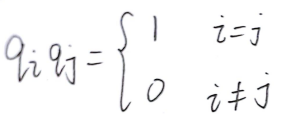标准指的是标准长度
假设A的列不是标准正交的，我们怎么把它变成标准正交的
A→Q
Q=[q1 q2 …qn]
利用上面的性质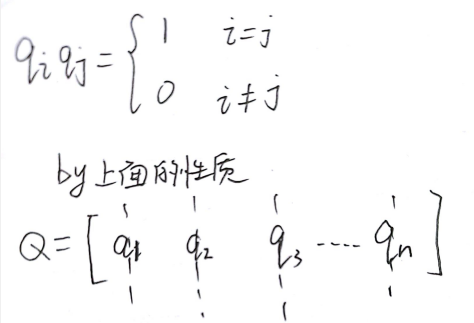让我们看一下QTQ
我们已经看过ATA，自然要看看QTQ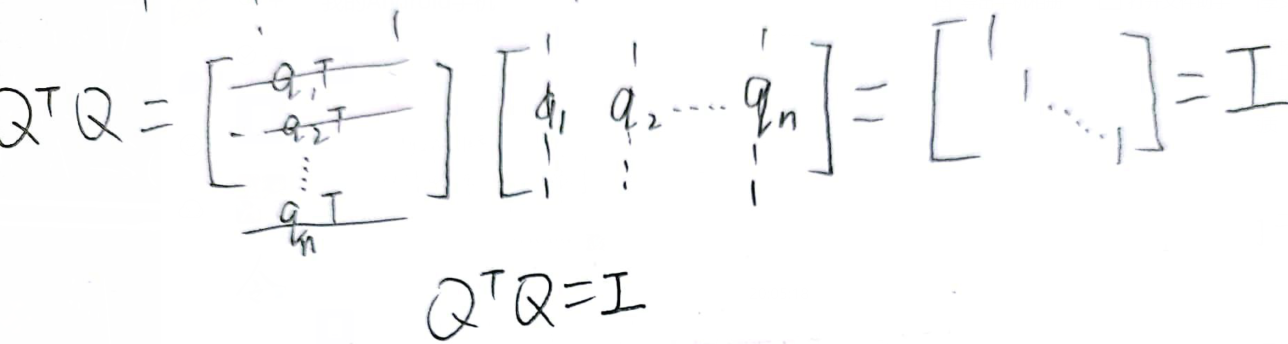甚至矩阵可以不是方阵！
现在我们得到了一些新的重要的矩阵
我们很久之前学过三角矩阵，对角矩阵，置换矩阵
行阶梯矩阵，投影矩阵，
现在学习标准正交矩阵
当矩阵是方阵的时候，我们称它为正交矩阵
为什么呢？
因为当Q为方阵，那么QTQ=I,then
QT=Q-1
这是标准正交的方阵的性质
让我们写点例子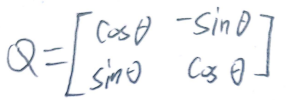再写个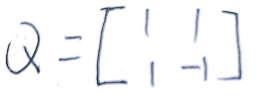注意，这个是正交矩阵吗？
No，长度不对，应该乘√2/2
最后举个大例子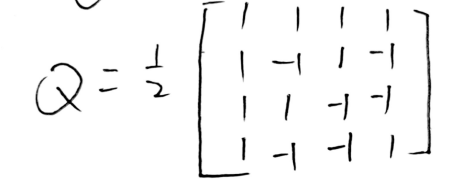我们学习了正交矩阵，它好在哪里？
格拉姆施密特正交化的缺点在于
因为这些向量必须是单位向量
所以很容易出现根号
那用Q有什么好处呢？那些公式会变得简单呢？
Q为正交矩阵，把他投影到列空间里
那它的投影矩阵是什么？
P=Q(QTQ)-1QT=QQT即是投影矩阵
假设矩阵是方阵？
且列向量标准正交
那么列向量就是整个空间！投影到自身
P=I
若不是方阵，结果就不是单位矩阵I了
投影矩阵的两条性质是什么？
首先它是对称的
第二条是（QQT）（QQT）=QQT
那很容易证明
之前所有的方程，如果Q替代A那就很容易了
最麻烦的ATAx=ATb
Now A is  Q
则上时变为x=QTb
这是个很重要的式子
如果我们知道标准正交基
在第i个基的投影就等于qib
后半节课
我们不在讨论正交矩阵
我们从线性无关向量开始，将其正交化
它不像消元法，因为我们的目标不是三角矩阵
从向量a，b开始
我想正交化它们，从中得到q1和q2
格莱姆和施密特提供了方法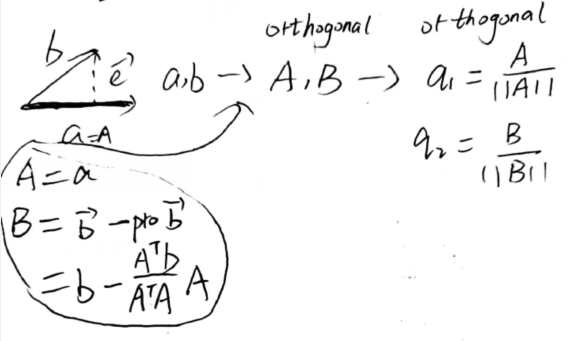下面再来讲求解和a、b正交的第三个向量c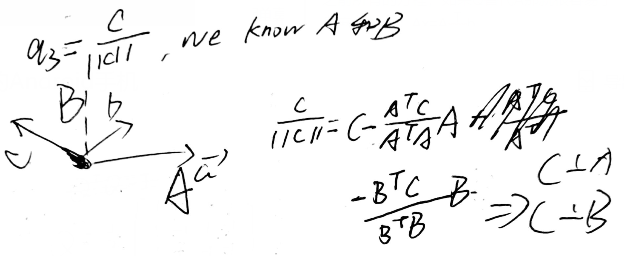举个例子
a=[1;1;1]
b=[1;0;2]
a=A‘=[1 ;1 ;1]
B=[1;0;2]- kA=[0;-1;1]
A=[1 0;1 -1;1 1]
Q=[q1 q2]=[1/√3 0;1/√3 -1/√2; 1/√3 1/√2]
回过头来用矩阵方法审视消元法
A=LU
已知A  Q
可以得到A=QR
即施密特格拉姆表达式
A和Q有相同列空间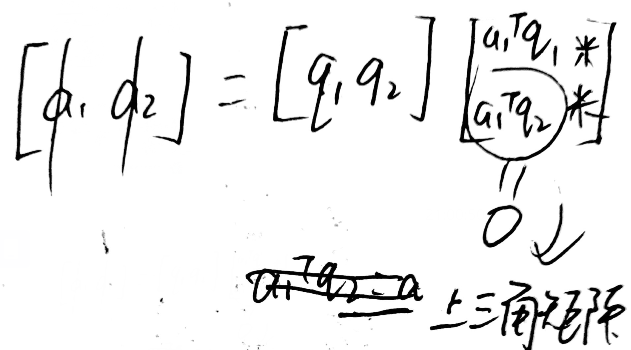任何矩阵都可以QR分解
一个列向量线性无关的矩阵A
格拉姆施密特方法构造正交矩阵Q
然后上三角矩阵R把它们联系起来
对于A=QR有另一种理解方式
比如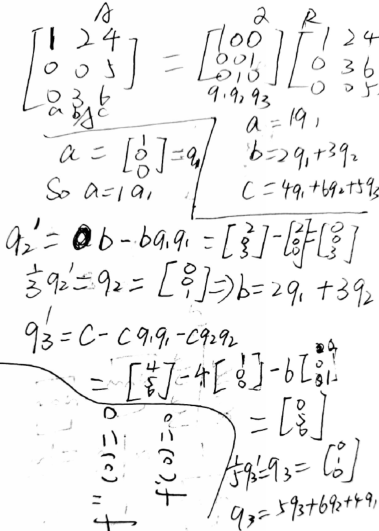展开全文• 最后我们得到正交化矩阵 Q = [ q 1 q 2 q 3 ] Q=\begin{bmatrix}q_1&q_2&q_3\end{bmatrix} Q = [ q 1 ​ ​ q 2 ​ ​ q 3 ​ ​ ] 实际上，并没有什么特殊的地方，这个正交化的过程就是逐步减去在已有基...
主要内容

标准正交基
正交矩阵
施密特正交化法

正文
标准正交基
先从字面意义上解读一下，然后再给出几个示例。正交意味着垂直，所以正交基就是说这一个基中的所有向量都是垂直的。而标准意味着，这组基中的向量都是单位向量，长度都为1。所以标准正交基实际上就是一个标准正交向量组。例如：$\begin{bmatrix}1\\0\\0\end{bmatrix}\begin{bmatrix}0\\1\\0\end{bmatrix}\begin{bmatrix}0\\0\\1\end{bmatrix}$ $\begin{bmatrix}cos\theta\\sin\theta\end{bmatrix}\begin{bmatrix}-sin\theta\\cos\theta\end{bmatrix}$ 使用数学公式表达这个定义为：$q^T_iq_j=\begin{cases}0\qquad if&i\ne j \\1\qquad if&i=j\end{cases}$
正交矩阵
实际上正交矩阵是一个简称，它的全称是标准正交方阵，它要求矩阵不仅是标准正交矩阵还得是方阵。
像上面的那个例子，我们将三个标准正交向量也就是一个标准正交向量组放到一个矩阵中，在这个矩阵中，每两列都是正交的，并且每列的长度都是$1$，该矩阵显然是一个方阵，所以这个矩阵就是一个正交矩阵。如下：$\begin{bmatrix}1&0&0\\0&1&0\\0&0&1\end{bmatrix}$现在我们将这个矩阵记为$Q$，他是由一组标准正交基$q$组成的：$Q=\begin{bmatrix}:&&:\\q_1&&q_n\\:&&:\end{bmatrix}$我们可以发现$Q^TQ=I$。这里就不给出证明了。由$Q^{-1}Q=I$，再结合前面的式子我们可以知道$Q^{-1}=Q^T$，这是正交矩阵特有的性质。
作用： 不知道正交矩阵是在研究投影的过程中产生的还是在什么时候产生的，但是它方便了投影上的计算。最重要的地方在于正交矩阵具有一个特殊的性质$Q^TQ=I$，这个性质对于所有的$Q$都是成立的，而不限制$Q$是否是方阵或者是长方形矩阵。对于$Ax=b$，我们知道，投影矩阵为$P=A(A^TA)^{-1}A^T$我们通过标准正交化，将$A$化成$Q$之后，再使用这个公式：$P=Q(Q^TQ)^{-1}Q^T=QQ^T$也就是说，正交矩阵简化了这个运算，使用正交矩阵计算投影矩阵的时候，我们会发现不用再求逆，而求逆是非常耗费计算量的，所以它应该是大大的减少了计算量。对于方阵而言， 我们可以得到$QQ^T=I$，即$P=I$。这是代数上的表示，在几何上分析可以发现：一个正交的方阵，也就是矩阵的所有列都是线性无关的，它的列空间就是整个空间。空间中的某个向量在向列空间上投影时，就是在向整个空间投影，得到的仍然是原来的位置。这就是$P=I$的原因。$\hat{x}=(A^TA)^{-1}A^Tb$ 现在$A$化成了$Q$，所以我们有下面的式子：$\hat{x}=(Q^TQ)^{-1}Q^Tb=Q^Tb$
施密特正交化法
正交矩阵简化了投影运算，于是如何将$A$转变成$Q$就成了问题，这就要用到Gram-Schmidt正交化法。
先分析一下简单的情况：两个线性无关的向量$a$，$b$，我们想要得到它们的一组标准正交基。之前我们学习过的投影保留列空间中的分量，而扔掉垂直于列空间的分量。相反，这次我们想保留垂直于列空间的分量，因为我们要找的就是垂直。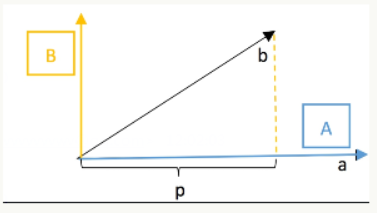像这种情况，我们想保留分量$B$，扔掉分量$p$。（这里$A=a$，因为我们将$a$作为第一个基$A$）$B=b-p$而且根据之前的内容，我们可以得$p=\frac{A^Tb}{A^TA}A$，带入到上式中可得：$B=b-\frac{A^Tb}{A^TA}A$至此我们得到了$A,B$，然后再将他们标准化：$q_1=\frac{A}{||A||}\qquad q_2=\frac{B}{||B||}$ $Q=\begin{bmatrix}q_1&q_2\end{bmatrix}$
然后我们将这种方法扩展到$3$维的情况，在进一步扩展到高维的情况时，跟这种扩展方法是类似的。有三个线性无关的向量$a,b,c$如图：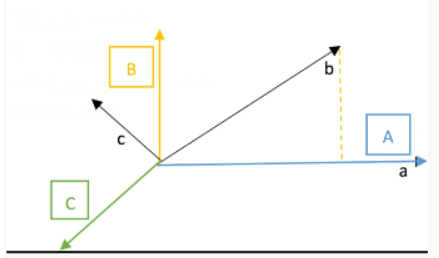我们仍然保留$a$做第一个基，类似前面的方法，$b$减去在$A$上的分量，保留垂直分量，做第二个基。$B=b-\frac{A^Tb}{A^TA}A$采用同样的思想，$c$减去在$A,B$上的分量，保留垂直于它们的分量做第三个基：$C=c-\frac{A^Tc}{A^TA}A-\frac{B^Tc}{B^TB}B$然后我们再标准化：$q_1=\frac{A}{||A||}\qquad q_2=\frac{B}{||B||}\qquad q_3=\frac{C}{||C||}$最后我们得到正交化矩阵$Q=\begin{bmatrix}q_1&q_2&q_3\end{bmatrix}$ 实际上，并没有什么特殊的地方，这个正交化的过程就是逐步减去在已有基上的分量的过程，最终保留了垂直于已有基的分量作为新的基。
在矩阵的角度上分析： 消元法的本质在于$A=LU$，即$A$的$LU$分解。这里的正交化法同样也可以写成类似的形式：$A=QR$右乘一个矩阵表示对$Q$进行列的线性组合，也就是说$A$是由它所在的空间中的基线性组合得到的。这是符合我们的常识的。


展开全文• 今天晚上王小民同学问了助教姐姐一个问题，为什么一个一般的矩阵对的时候，我们不用做正交单位实对称矩阵对的时候却要做呢？这是一个很好的问题，所以和大家分享一下。 最后的结论就是...
今天晚上王小民同学问了助教姐姐一个问题，为什么对一个一般的矩阵对角化的时候，我们不用做正交单位化，对实对称矩阵对角化的时候却要做呢？这是一个很好的问题，所以和大家分享一下。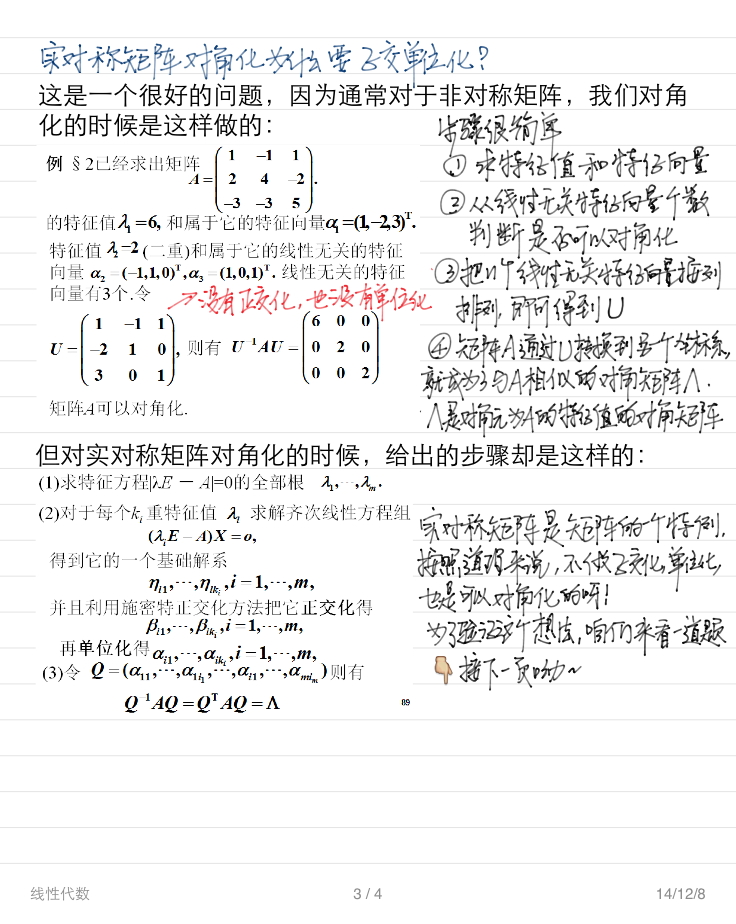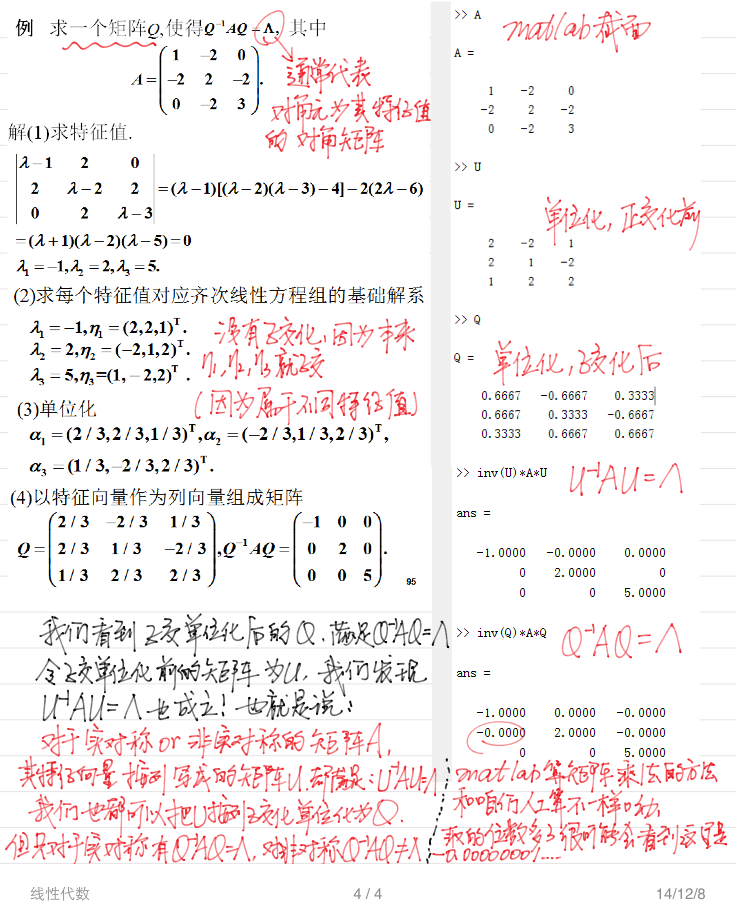最后的结论就是：如果不做正交单位话，我们一样可以通过U（把特征向量按照列写成的矩阵），把一个实对称矩阵对角化为以它的特征值为对角元的对角矩阵。

我们知道，对应一个特征值的特征向量乘以任何一个非零的系数，仍然还是对应着这个特征值的特征向量，如果一个特征值对应多个特征向量，那在它们张成的空间里找出同样数量的线性不相关的向量，也都是这个特征值的特征向量，所以说特征向量并不唯一，也就是说这里的U是不唯一的。

而对于一个实对称矩阵，它的属于不同特征值的特征向量天生就是正交的，这使得我们只要在每个特征值内部选取合适的互相正交的特征向量，就能保证所有的特征向量都正交。而我们刚刚说过，特征向量乘以一个系数，仍然还是特征向量。所以，对于实对称矩阵来说，我们完全可以在诸多的U中选出一个特殊的Q，让Q的每一个列向量都互相正交而且长度为1。这时我们就惊喜的发现，这样的相当于由一组标准正交基当做列向量组成的矩阵Q，正是一个正交矩阵。

于是，我们就清楚的知道了，对实对称矩阵对角化的时候，正交单位化不是必须的，只有当我们想在实对称矩阵的诸多U里选取一个正交矩阵Q时，才需要做。正交矩阵有很多很好的性质，于是乎想从U里找到一个Q也变得情有可原了不是？

展开全文• 矩阵论施密特正交化，通俗释义。 已知空间向量γ，α，β，求取，目标将α正交化至β方向上。 1、α在γ方向上的投影s为： 2、α在γ方向上的分量为： s乘以γ方向上的单位向量： 即式子为： 3、消除...
矩阵论施密特正交化，通俗释义。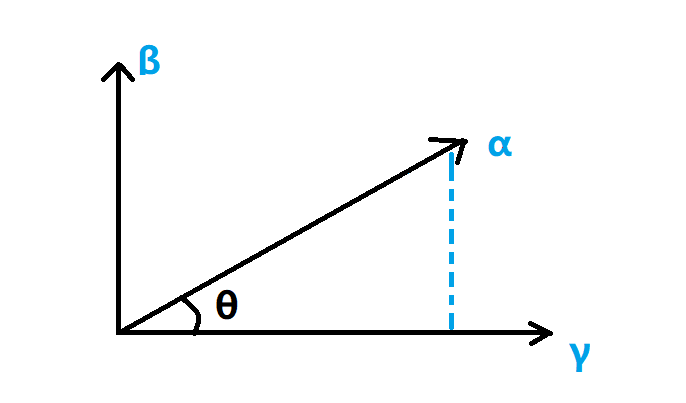已知空间向量γ，α，β，求取，目标将α正交化至β方向上。

1、α在γ方向上的投影s为：

2、α在γ方向上的分量为：

s乘以γ方向上的单位向量：

即式子为：

3、消除α在γ方向上的分量,进而得到了α在β方向上的分量：

综上，完成了一次施密特正交化。

附加：

如果拓展成高维度，比如三维，即有三个基向量，即三个方向的维度，那么求取三维空间在某一维度的分量，需要该空间向量减去另外两维度的分量。往往正交化的过程是先将某一个向量设定为第一个维度的向量，后面的向量依次进行正交化，当然要消除前一维度的分量。


展开全文• 写在word里面，我截图放上来吧 目录 ...施密特正交化： 施密特正交化例题： 向量的内积： 正交、正交矩阵： 规范正交向量组： 施密特正交化： 施密特正交化例题： ...
• n阶矩阵A可正交对的充分条件是A是实对称矩阵，即若A是实对称矩阵则A必可正交对。 首先，有以下定理： 若的特征值为，且，则存在正交矩阵Q，使A相似于如下三角矩阵： 证明如下（数学归纳法）： 设n*n阶...
• 什么是正交矩阵和Gram-Schmidt正交化,相信学过线性代数的朋友们都知道. 这里,我只想说标准正交化和标准正交矩阵带来的好处. 标准正交化(orthonormal)的定义是:如果向量q1,q2,...,qn满足下式 则q1,q2,matrix
• 题目：对称矩阵、Hermite矩阵正交矩阵、酉矩阵、奇异矩阵、正规矩阵、幂等矩阵  看文献的时候，经常见到各种各样矩阵，本篇总结了常见的对称矩阵、Hermite矩阵正交矩阵、酉矩阵、奇异矩阵、正规矩阵、幂等...
• 基于matlab软件，利用施密特正交化过程，对矩阵实现QR分解，得到正交矩阵Q和上三角矩阵R。
• 线性代数中最头疼的公式恐怕就是施密特正交化了。但其实搞清楚它的几何原理之后公式的记忆就简单多了，数学重在理解! 给定一组基α1,α2,...
• 1、标准正交矩阵  假设矩阵Q有列向量q1,q2,...,qn表示，且其列向量满足下式： 则 若Q为方阵，由上面的式子则有 我们举例说明上述概念： 2、标准正交矩阵的好处  上面我们介绍了标准正交矩阵，那么标准...正交向量 schmidt 线性代数
• 文章目录实对称矩阵对称变换实对称矩阵的正交参考 本节目的: 讨论一类必可相似的矩阵: 实对称矩阵. ...给出实对称矩阵正交对的方法. 实对称矩阵 复数及其性质: i2=−1,i:\quad \mathbf{i}^{2}=-
• 一是了解正交性是怎么让 x^\hat xx^ 、ppp 、PPP 的计算变得简单的，这种情况下，ATAA^TAATA 将会是一个矩阵。二是学会怎么从原始向量中构建出正交向量。 1. 标准正交基 向量 q1,⋯&amp;ThinSpace;,qnq_1, ...
• 正交定义 正交例题 正交向量组 标准正交基 Schmidt正交化 例题
• 第十七课时：正交矩阵和Gram-Schmidt正交化 这是关于正交性最后一讲，已经知道正交空间，比如行空间和零空间，今天主要看正交基和正交矩阵 一组基里的向量，任意q都和其他q正交，两两垂直，内积为零，且qi不...标准正交基
• 今天来谈谈正交矩阵和Gram-Schmidt正交化。 先来看看正交矩阵正交矩阵在详细讨论正交矩阵之前， 我们先来看看正交向量。 假设q1, q2……qn 是一组正交向量， 那么每一个q向量都和其他的q向量正交（垂直）。 也就是说...
• 在求解涉及旋转的优化问题过程中，通过解线性方程组或者采用优化方法得到的旋转矩阵的数值解，往往没有考虑到正交约束，会得到一个存在误差的近似旋转矩阵，也就是说这个矩阵并不是一个严格正交的标准正交矩阵，不...
• 这是关于正交性最后一讲，已经知道正交空间，比如行空间和零空间，今天主要看正交基和正交矩阵 1.标准正交基与正交矩阵 1.定义标准正交向量（orthonormal）： qTiqj={01i!=ji=jqiTqj={0i!=j1i=jq^T_iq_j= \be.....线性代数
• 1、归一： 首先按行归一： % Examples A=[3 4;5 12]; [m n] = size(A); % normalize each row to unit for i = 1:m  A(i,:)=A(i,:)/norm(A(i,:)); end  按列...函数 matlab
• ## 正交矩阵

千次阅读 2019-02-23 15:37:23
• 【功能简介】将矩阵正交规范，得到矩阵的正交基。 【语法格式】 B=orth(A)  返回矩阵A的正交基，B的列与A的列具有相同的空间，B的列向量是正交向量，满足B'*B = eye(rank(A))，B的列数是A的秩。 【实例...
• ）或A^TA=E，则n阶实矩阵A称为正交矩阵，若A为正交阵，则满足以下条件 : 1)A^T是正交矩阵 2)E为单位矩阵 3)A的各行是单位向量且两两正交 4)A的各列是单位向量且两两正交 5)(Ax,Ay)=(x,y) x,y∈R ......